Next: Generalities of Track Fitting Up: General Discussions without Momentum Previous: General Discussions without Momentum

# Helix Parametrization

A charged particle in a uniform magnetic field follows a helical trajectory. In what follows we set our reference frame so that it has its z axis in the direction of the magnetic field. There are many ways to parametrize this helix. The following choice, however, greatly facilitates various operations we are going to make on tracks, such as track extrapolation and linking: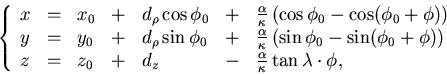(1)
where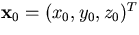specifies an arbitrarily chosen pivotal point or simply pivot: we usually take as a pivot a hit or wire position of the tracking device or a position of track linking. Once the pivotal point is fixed, the helix is determined by a 5-component parameter vector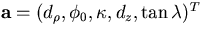, where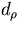is the distance of the helix from the pivotal point in the xy plane,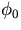is the azimuthal angle to specifies the pivotal point with respect to the helix center,is the signed reciprocal transverse momentum so that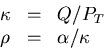with Q being the charge,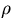being the signed radius of the helix, and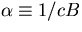being a magnetic-field-dependent constant, dz is the distrance of the helix from the pivotal point in the z direction, andis the dip angle. The deflection angle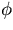is measured from the pivotal point and specifies the position of the charged particle on the helical track. The meanings of these parameters are depicted in Figs.2.1-a) for negatively charged and -b) for positively charged particles.Notice that a negatively charged particle travels in the increasingdirection, while a positively charged particle travels in the decreasingdirection.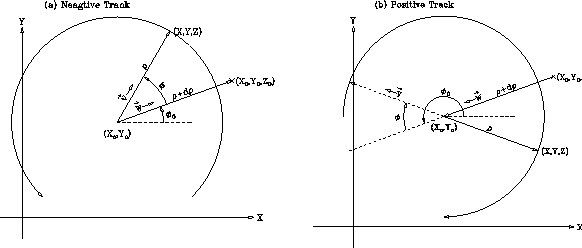Taking this into account it is easy to get the following formula which relates the momentum of a charged particle to its helix parameters: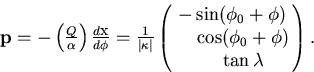(2)Next: Generalities of Track Fitting Up: General Discussions without Momentum Previous: General Discussions without Momentum
Keisuke Fujii
12/4/1998